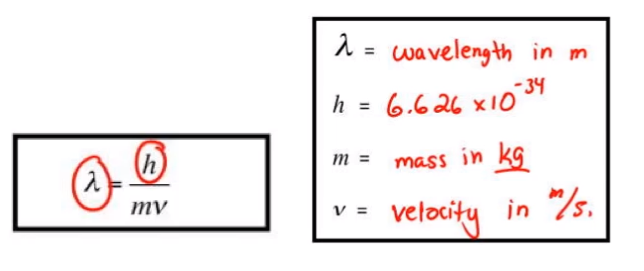Ch.7 - Quantum MechanicsWorksheetSee all chapters
 Ch.1 - Intro to General Chemistry 2hrs & 53mins 0% complete Worksheet Ch.2 - Atoms & Elements 2hrs & 40mins 0% complete Worksheet Ch.3 - Chemical Reactions 3hrs & 25mins 0% complete Worksheet BONUS: Lab Techniques and Procedures 1hr & 38mins 0% complete Worksheet BONUS: Mathematical Operations and Functions 47mins 0% complete Worksheet Ch.4 - Chemical Quantities & Aqueous Reactions 3hrs & 30mins 0% complete Worksheet Ch.5 - Gases 3hrs & 47mins 0% complete Worksheet Ch.6 - Thermochemistry 2hrs & 28mins 0% complete Worksheet Ch.7 - Quantum Mechanics 2hrs & 35mins 0% complete Worksheet Ch.8 - Periodic Properties of the Elements 1hr & 57mins 0% complete Worksheet Ch.9 - Bonding & Molecular Structure 2hrs & 5mins 0% complete Worksheet Ch.10 - Molecular Shapes & Valence Bond Theory 1hr & 31mins 0% complete Worksheet Ch.11 - Liquids, Solids & Intermolecular Forces 3hrs & 40mins 0% complete Worksheet Ch.12 - Solutions 2hrs & 17mins 0% complete Worksheet Ch.13 - Chemical Kinetics 2hrs & 22mins 0% complete Worksheet Ch.14 - Chemical Equilibrium 2hrs & 26mins 0% complete Worksheet Ch.15 - Acid and Base Equilibrium 4hrs & 42mins 0% complete Worksheet Ch.16 - Aqueous Equilibrium 3hrs & 48mins 0% complete Worksheet Ch. 17 - Chemical Thermodynamics 1hr & 44mins 0% complete Worksheet Ch.18 - Electrochemistry 2hrs & 58mins 0% complete Worksheet Ch.19 - Nuclear Chemistry 1hr & 33mins 0% complete Worksheet Ch.20 - Organic Chemistry 3hrs 0% complete Worksheet Ch.22 - Chemistry of the Nonmetals 2hrs & 1min 0% complete Worksheet Ch.23 - Transition Metals and Coordination Compounds 1hr & 54mins 0% complete Worksheet

# De Broglie Wavelength

See all sections

The De Broglie Wavelength equation relates wavelength to velocity or speed.

###### Wave Nature of Light

Concept #1: The De Broglie Wavelength Equation

Transcript

In this new video, we're going to take a look at the wave nature of light. Now, we've talked about light being composed of small packets of electromagnetic energy. We say that each one of these packets of this energy were called quantums way long ago. In modern days now, we call them photons, so we say light is composed of small particles called photons, but we've also talked about the inverse relationships of frequency and wavelength. In those examples, we've talked about light moving as a wave.
In this section, we're going to stick to that type of idea. We're going to see light as moving not as individualized particles but rather as a wave. We're going to say there's an equation that shares this relationship of light moving as a wave. It's called de Broglie wavelength equation. Under this equation, it says light moves as a wave, not as individual photons, individual particles. Now, we're going to say to calculate the wavelength of matter, we simply use this equation.
Now, the equation is for de Broglie wavelength equation is, lambda which is our wavelength equals h which is Planck's constant divided by m times v. We know that lambda is wavelength in meters. H is Planck's constant which we've talked about. Here m represents the mass of the object we're talking about. Usually, this object is subatomic particle. Here it will be the mass in kilograms, not in grams. v does not represent our frequency anymore. V here is actually velocity or speed. Velocity or speed are in meters per second.
What we need to realize here about Planck's constant is Planck's constant is 6.626 x 10-34 joules times seconds. We should realize that joules can be written a different way. Joules also equal kilograms times meters squared over seconds squared. That's what joules equal. If our joules are still multiplying with those seconds, then seconds will be canceled out with one of the seconds here.
In this equation, we're going to say Planck's constant becomes 6.626 x 10-34 kilograms times meters squared over seconds. Just realize how those things canceled out to give me that. Those are the units that you're going to have to remember when it deals with de Broglie wavelength.Example #1: Find the wavelength (in nm) of a proton with a speed of 7.33 x 109 . (Mass of a  proton = 1.67 x 10-27 kg)

Practice: What is the speed of an electron that has a wavelength of 895 μm? (Mass of an electron = 9.11 x 10 -31 kg)